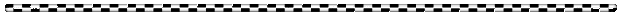# Metric System

Metric System (SI)Scientist use a single, standard system of measurement.  The official name of the measurement system is SYSTEME INTERNATIONAL d’UNITES (International System of Measurements) or SI.

The metric system is based on the number 10.

 Main Units of Measurement Length Volume Mass meter (m) liter (l) gram (g)

Using the above values (meter, liter, & gram) as the base, their value can be increased or decreased by moving the decimal point to the right (lowers the value) or left (raises the value).

## Metric Conversion Table

Kilo-
(k)
Base Unit
(m, l, g)
Centi-
(c)
Milli-
(m)
Micro-
(µ)
nano-
(n)
x 1000meter, gram, liter100100010001000
10001.01.001.000001.000000001

Convert the following values by moving the decimal point the correct number of spaces and in the right direction .

1. 69.8 meters (m) =  ________________ centimeters (cm)

2. 152.97 milliliters (ml)  =  ________________ liters (l)

3. 42.67 liters (l) = _____________ milliliters (ml)

4. 299.32 kilometers (km) = ____________ nanometers (nm)

5. 26 grams (g) = _____________ kilograms (kg)

6. 123.43 centigrams (cg) = ______________ grams (g)

7. 75.2 liters (l)  = __________________milliliters (ml)

8. 456.3 grams (g) = ________________ micrograms µg

9. 4507.22 kilometers (km) = _______________millimeters (mm)

10. 0.00297456 kilograms (kg) = ___________ nanograms (ng)

 BACK